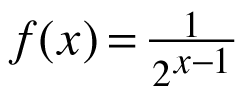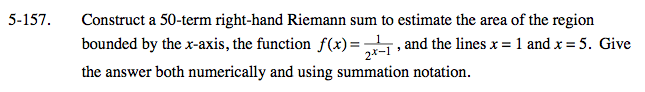### Home > CALC > Chapter 5 > Lesson 5.5.1 > Problem5-157

5-157.

Construct a 50-term right-hand Riemann sum to estimate the area of the region bounded by the x-axis, the function, and the lines x = 1 and x = 5. Give the answer both numerically and using summation notation. Homework Help ✎General form of a right-endpoint Riemann sum: